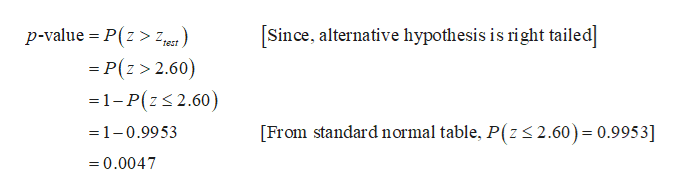# A test is made of H0: u= 50 versus H1: u is greater than 50. A sample of size n=75 is drawn, and mean = 56. The population deviation is"q"=20.a. Compute the value of the test statistic z.b. Is H0 rejected at the a=0.05 level?c. Is H0 rejected at the  a=0.01 level?

Question
376 views

A test is made of H0: u= 50 versus H1: u is greater than 50. A sample of size n=75 is drawn, and mean = 56. The population deviation is"q"=20.

a. Compute the value of the test statistic z.

b. Is H0 rejected at the a=0.05 level?

c. Is H0 rejected at the  a=0.01 level?

check_circle

Step 1

a)

The value of the test statistic is 2.598 and it is obtained below:

From the given information, the sample size is 75, mean is 56 and the population standard deviation is 20.

Null Hypothesis: H0: µ=50.

Alternative Hypothesis: H1: µ>50.

Step 2

b)

Decision Rule:

If p-value ≤ α, then reject the null hypothesis.

If p-value > α, then do not reject the null hypothesis.

The p-value for the hypothesis testing is 0.0047 and it is obtained below:help_outlineImage Transcriptionclose[Since, alternative hypothesis is right tailed] p-value P(z > est) -P(z> 2.60) 1-P(z2.60) 2.60) = 0.995 3] From standard normal table, P(z =1-0.9953 =0.0047 fullscreen
Step 3

Conclusion:

From the given information, the level of significance is α=0.05

The p-value is 0.0047.

Here, the p-value is less than the level of significance.

That is, p(=0.0047) < α=0.05.

From the rejecti...

### Want to see the full answer?

See Solution

#### Want to see this answer and more?

Solutions are written by subject experts who are available 24/7. Questions are typically answered within 1 hour.*

See Solution
*Response times may vary by subject and question.
Tagged in
MathStatistics

### Hypothesis Testing